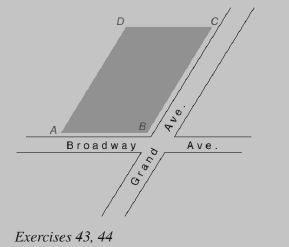Chapter 4.1, Problem 44EElementary Geometry For College St...

7th Edition
Alexander + 2 others
ISBN: 9781337614085

Solutions

Chapter
SectionElementary Geometry For College St...

7th Edition
Alexander + 2 others
ISBN: 9781337614085
Textbook Problem

To test the accuracy of the foundation’s measurements, lines (strings) are joined from opposite corners of the building’s foundation. How should the strings that are represented by A C - and B D - be related?To determine

To find:

The relationship between the diagonals represented by AC- and BD-.

Explanation

Given:

Two streets meet to form an obtuse angle at point B. On that corner the newly poured foundation for a building takes the shape of a parallelogram.

Corollary:

The diagonals of a parallelogram bisect each other.

Approach:

In the given parallelogram ABCD, AC- and BD- are diagonals

Still sussing out bartleby?

Check out a sample textbook solution.

See a sample solution

The Solution to Your Study Problems

Bartleby provides explanations to thousands of textbook problems written by our experts, many with advanced degrees!

Get Started

In Exercises 1-4, simplify the expression. x2+6x+8x26x16

Calculus: An Applied Approach (MindTap Course List)

Other Equations Find all real solutions of the equation. 102. 1+x+1x=2

Precalculus: Mathematics for Calculus (Standalone Book)

Find the mean, median, and mode for the following scores; 8, 7, 5, 7, 0, 10, 2, 4, 11, 7, 8, 7

Essentials of Statistics for The Behavioral Sciences (MindTap Course List)

Prove the identity. 48. tan2 sin2 = tan2 sin2

Single Variable Calculus: Early Transcendentals

The graph of is: a) b) c) d)

Study Guide for Stewart's Multivariable Calculus, 8th

Using tan2 x = sec2 x − 1, ∫ tan3 x dx =

Study Guide for Stewart's Single Variable Calculus: Early Transcendentals, 8th